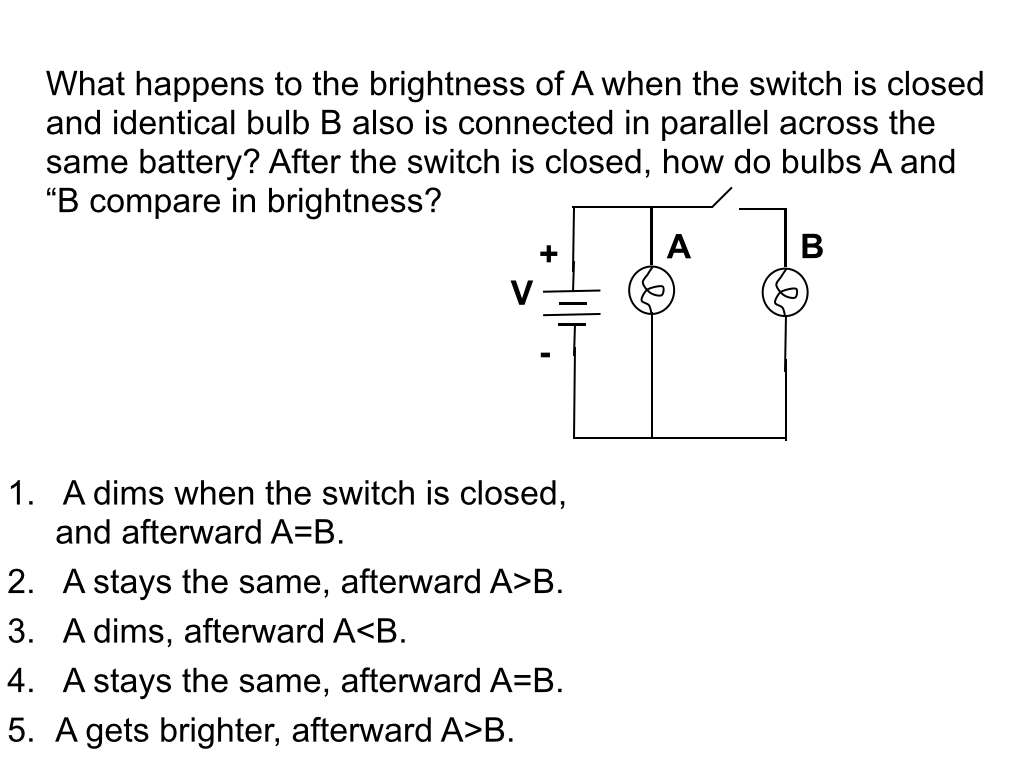# Day 100: Circuits Peer Instruction

My AP Physics B students can play circuit sudoku and analyze fairly sophisticated DC circuits. Once I take away all the numbers and they have to reason through the problem conceptually, they are challenged. This is another example of where peer instruction can be effective. Students tend to favor analyzing circuits from a current model rather than a voltage model and, therefore, struggle with problems like this one:As groups discussed this question, I heard the expected claim: “When the switch is closed, the current is now split between A and B; so, A will be dimmer.” The first part of this statement is true but not the second as the students are assuming constant current. After some discussion, students questioned each other enough to shift to the voltage model and realize that the potential difference across A would not change. Certainly a better use of class time than another circuit sudoku practice problem!

##circuits